Fast Mental Math
Geometry
Algebraic Manipulation
Word Problems**
Graphs

100

The number that is 16% of 25.

100

The value of the angle in red.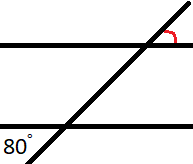100

The isolated form of T in the equation provided.

K=3/2kT

100

Jim had 28 apples. He then gave away 4 of them. Afterwards he lost half of his apples so Jill gave him 3 apples. How many apples does Jim have now?

100

The number of times the line x=3 intersects with the graph provided.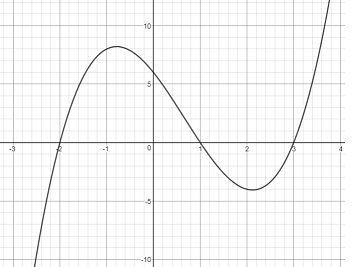200

The value when 8 increased by 200%.

200

The value of z.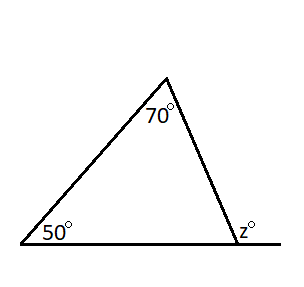200

The positive expression for r in the equation provided.

F_G=(Gm_1m_2)/r^2

200

If a car rental agency charges $50 to take the car out the door plus an additional$22 at the end of the day, this would be the y-intercept of the cost graphed versus time.

200

The number of times y=2x intersects with the equation provided on the graph.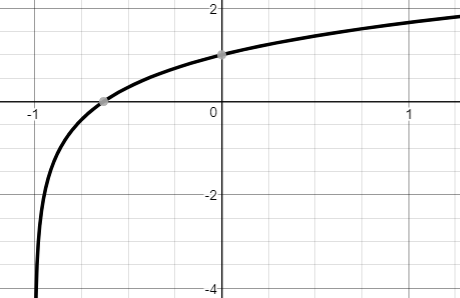300

A number increased by 20% is 216.

300

The measure of angle x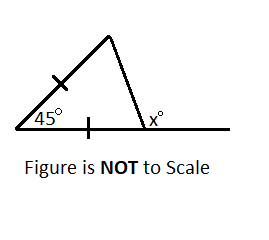300

Solve for x0 in the equation provided.

v^2=v_0^2+2a(x-x_0)

300

A chemist has 2L of solution that is 40% acetic acid. She also has a reservoir of acid that is 10%. How many liters should she add from the reservoir to the 2L of 40% solution to make a solution that is 15%?

300

When graphed, this equation makes this shape.

x^2+2x+y^2+5=17

400

50 is this percent of 40.

400

The area of the figure in terms of integers and

pi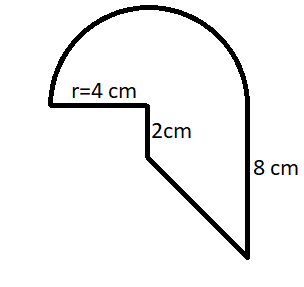Solve for 'x'.

x^2=sqrt(81)

400

Lara is 84% the height of Brandon. If Paul is 25% taller than Lara and Brandon is 75 inches tall, how tall is Paul?

400

The number of unique roots of the product of the two functions graphed.163/5

as a decimal.

500

The area of a regular hexagon with a side measuring 6 cm.

500

The provided equation shows the energy of an object that is launched from a spring on an elevated surface that eventually hits the ground at a certain velocity. If 'x' is the positive compression of the spring, solve for how far compressed the spring was.

1/2kx^2+mgh=1/2mv^2

500

It takes 3 painters 40 minutes to paint a small room and 5 painters 50 minutes to paint a big room. How many hours does it take for 7 painters to paint 5 small rooms and 6 big rooms?

500

When graphing polynomials on the real plane, this is the amount of imaginary intercepts that can be observed.